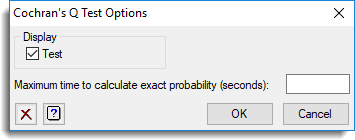1. Home
2. Cochran’s Q Test Options

# Cochran’s Q Test Options

Use this to set options for Cochran’s Q test.## Display

Specifies the output to be displayed.

 Test The Q value and probability.

## Maximum time to calculate exact probability

Provides a space to specify, in seconds, the maximum time to allow for the calculation of the exact probability. Although the menu uses an efficient algorithm for calculating the exact probability, the time and memory required for this calculation can become impractical as the number of samples and values increases. Therefore, this option can be used to specify the maximum amount of time allowed for an exact calculation before the computation is terminated.